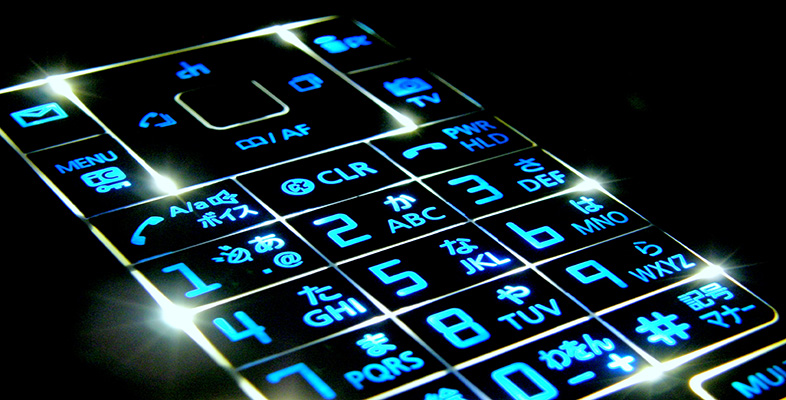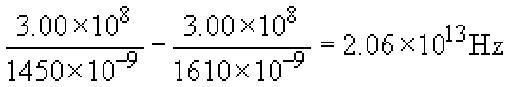Digital communications

Start this free course now. Just create an account and sign in. Enrol and complete the course for a free statement of participation or digital badge if available.

# 2.4 Pulse spreading and bandwidth

## Activity 3

Calculate the maximum signalling rate given by the Nyquist rate for the 1550 nm window, assuming that it runs from 1450 nm to 1610 nm.

Using the exact method from Section 1.2, the bandwidth is:So the Nyquist rate is 4.12 ×1013 baud, or about 41 Tbaud.

This activity accurately portrays the massive potential capacity of optical fibre, but in practice it is not possible to exploit the full capacity directly – binary modulation at terabaud rates is a long way off!

In practice, the usable signalling rates in fibre are limited either by the speed at which the electronics at the transmitter and receiver can operate (at the time of writing, state-of-the-art commercial systems operate at up to 40 Gbaud) or by effects in the fibre that cause pulses to spread as they propagate along the fibre. Pulse spreading limits the maximum signalling rate because the more that pulses spread the more inter-symbol interference (ISI) you can get, and so the further apart in time symbols must be to prevent them interfering with each other. The amount of pulse spreading is proportional to distance – if pulses are spread by 1 ps (picosecond, 10−12 s) when travelling through 1 km of fibre then they will spread 2 ps over 2 km – so there is a trade-off between the usable bandwidth of fibre and the fibre length.

## Actvity 4

Suppose a fibre spreads pulses at the rate of 200 ps km−1. What, approximately, is the maximum signalling rate that can be used with this fibre over a distance of: (a) 1 km, (b) 10 km?

Over 1 km the pulses spread by 200 ps and over 10 km they spread by 2 ns. You have to make some modelling assumptions about the pulses and at what point spreading will cause errors, but a starting point might be that the spreading must be less than half the symbol period, τ = 1/S, where S is the signalling rate. Over 1 km then, 0.5 × 1/S = 200 ps, so S = 0.0025 ×1012 = 2.5 Gbaud, and over 10 km we have S = 250 Mbaud.

A more complete analysis would take account of the fact that pulses don't remain rectangular as they spread, and that the effects are statistical – the error rate increases with increasing pulse spreading. The simple model of allowing spreading by up to half a symbol interval is adequate for order-of-magnitude considerations, however.

The main causes of pulse spreading differ between multimode and single-mode fibre, and will be considered next.